## Stacked Tetratans

Here you can see the set of the 66 tetratans,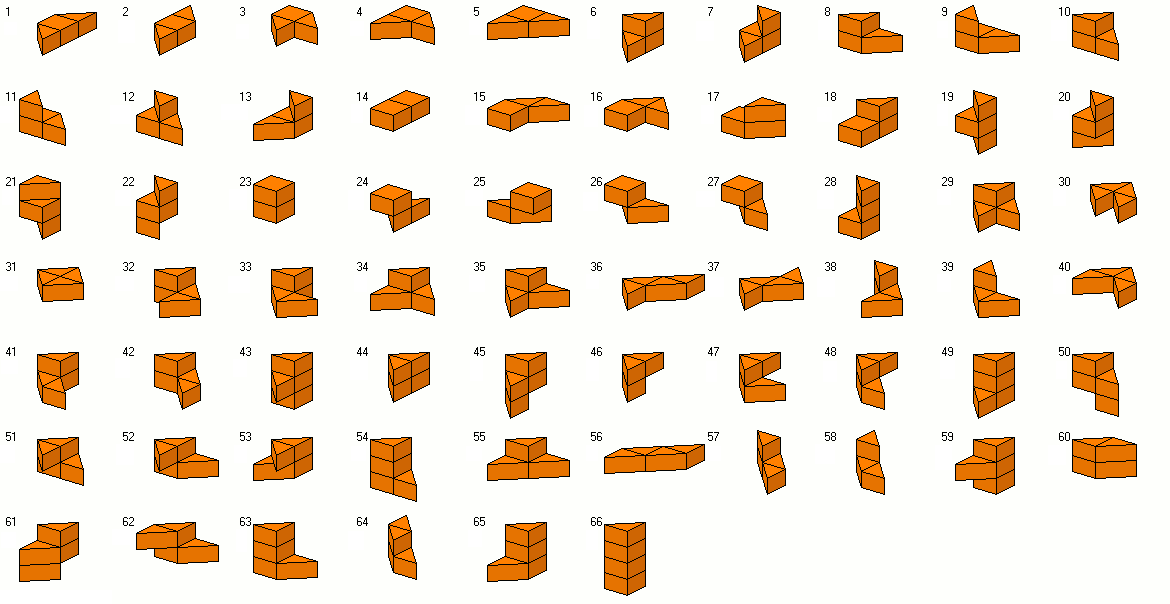But it's a real mess with these pieces. If you try to make some constructions with the whole set, you must pay attention to three constraints:
1. The number of triangles placed on black elements of a checkerboard colored box must be odd.
2. The number of all h-sides with negative slope, i.e. sides going from top left corners to bottom right corners, must be even.
3. The sum of triangles in all odd layers must be odd.
We can refer to these constraints by 'odd chequering', 'even slope' and 'odd stacking'.

With a total number of 66*4=264=2*2*2*33 prisms can't meet these conditions. If the number of layers is even, odd chequering is violated.If the number if layers is odd, each cross-section has 8 triangles and odd stacking is wrong.

I looked for symmetric construction with odd chequering, odd stackin and even slope and found kind of pyramids, that could be solved.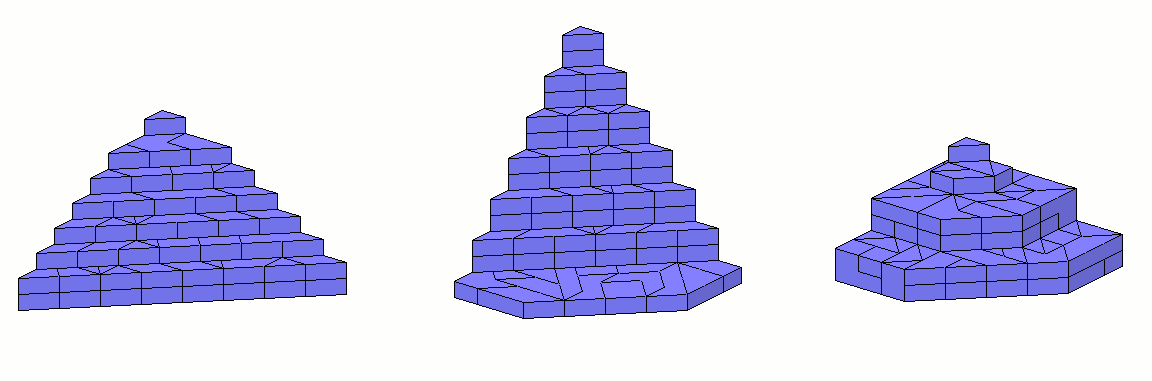The construction was split into two parts and during the search for the first part good pieces were saved for the second part. Here are the layers of the first the second and the third pyramid.

If we only use 65 pieces, a lot of prisms can be constructed. If the unused piece is symmetric with even slope, odd chequering und odd stacking, it can be put on symmetric prisms to get a symmetric construction with all pieces. There are only three pieces, that meet these conditions: #28, #29 and #55.

Since 65*4=2*2*5*13 we can look for prisms with height 4, 5, 10, 13, 20 or 26 and corresponding cross-sections of 65, 52, 26, 20, 13 or 10. Some examples with height 4, 10, 13, 20, a symmetric cross-sections and 8, 7, 5, 6 corners are shown. For height 26 only a non convex cross-section with no symmetry seems to be possible.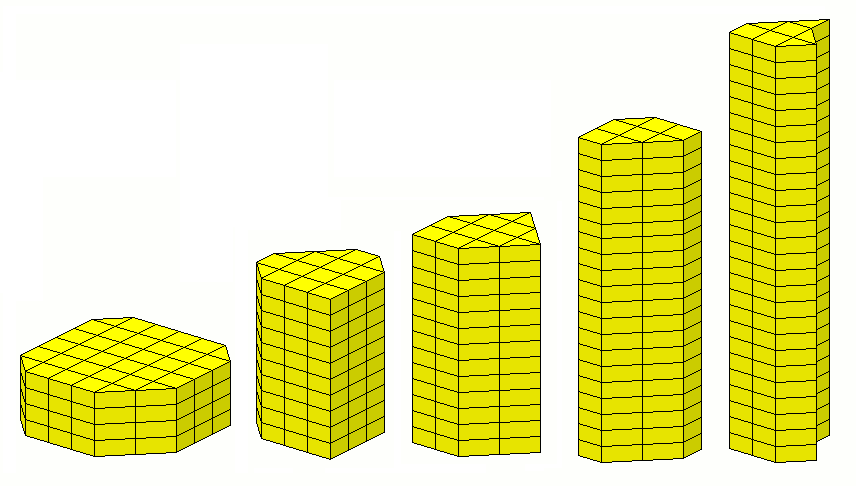The layers for these prisms are here.

For height=5 I made some prisms with rotational symmetry.The layers for these prisms are here.

If you aks for rectangular solids with 65 pieces you must check the following cases

 Height Number of Triangles in the Cross-Section Possible Rectangles 26 10 1s x 5s 20 13 no rectangle, because 13 is odd 13 20 2s x 5s or 1h x 5h 10 26 1s x 13 s 5 52 2s x 13s or 1h x 13h 4 65 no rectangle, because 65 is odd

Some pieces don't fit a rectangle with one side equal to 1s or 1h. Therefore such construction are impossible. The prism with the 2s x 5s bottom area and height =13 has a curved surface area of (2+5)*2*13 = 182. This is too much since the maximum contribution of all pieces to the vertical faces is about 174. The prism with the 2s x 13s bottom area and height=5 has a curved surface area of (2+13)*2*5*150 < 174 and might be the only possible box.
After adjusting the filling sequence and bound conditions in the search tree a solution was found.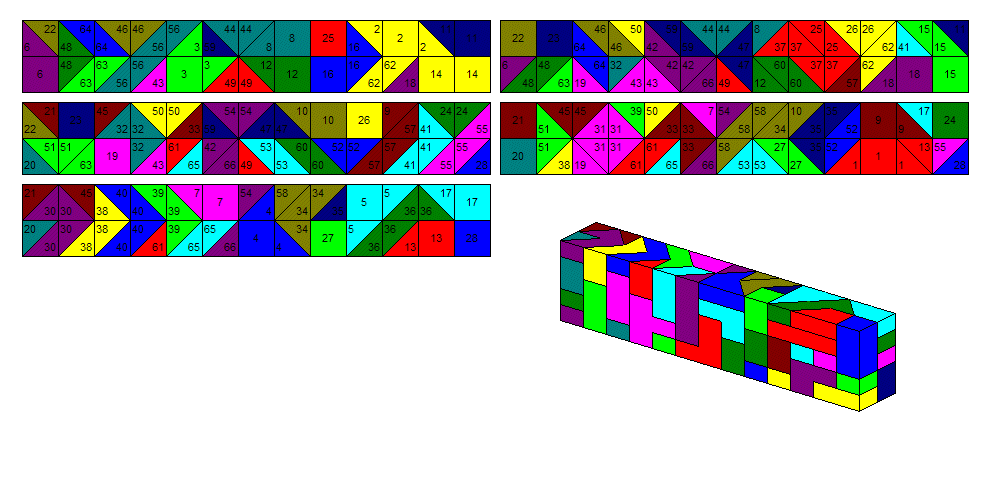At last I looked for 4-fold replicas of the pieces using only 64 pieces with two pieces left, perhaps one being the replicated one. These are too many problems and I decided to make two 4-fold replicas of a tan and with the remaining pieces 4-fold replicas of three different ditans. With combinations of these parts all pieces can be replicated.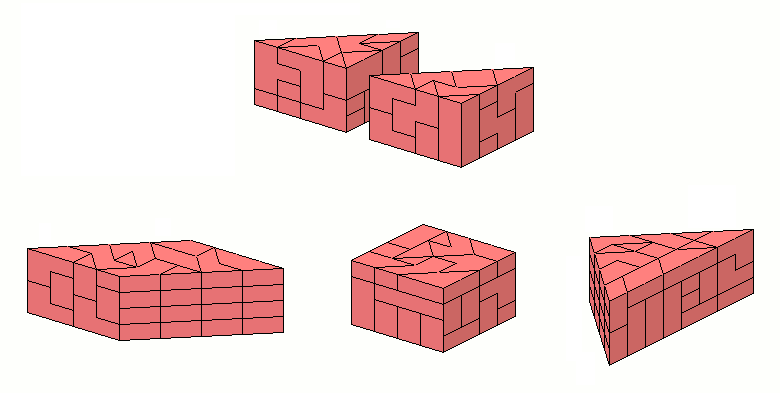The layers for these parts are here.

Back
Home